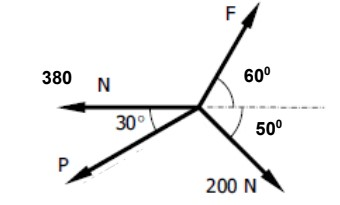# Determine the magnitude of P and F necessary to keep the concurrent force system in Fig. below in equilibrium.

Question-AnswerCategory: Engineering MechanicsDetermine the magnitude of P and F necessary to keep the concurrent force system in Fig. below in equilibrium.

## Determine the magnitude of P and F necessary to keep the concurrent force system in Fig. below in equilibrium.Concept:
If a system of three concurrent and coplanar forces is in equilibrium, then Lami’s theorem states that the magnitude of each force of the system is proportional to sine of the angle between the other two forces.F.Horizontal:-

ΣFV=0ΣFV=0
Fsin60=200sin45+Psin30Fsin⁡60∘=200sin⁡45∘+Psin⁡30∘
(317.16+1.7320P)sin60=200sin45+Psin30(317.16+1.7320P)sin⁡60∘=200sin⁡45∘+Psin⁡30∘
274.67+1.5P=141.42+0.5P274.67+1.5P=141.42+0.5P

F=317.16+1.7320(133.25)F=317.16+1.7320(−133.25)

*Solved by ~Atul / Sliet / GCT-2

F.Horizontal:-

F.Horizontal:-

→200sin315° + 300sin180° + Psin210°+ Fsin60°

→F= 2(158.6 + 0.866P)

→F= 317.2 + 1.732P

F.Vertical:-
Fsin60=200sin45+Psin30°
Now put the value of ‘F’
(317.16+1.7320P)sin⁡60∘=200sin⁡45∘+Psin⁡30∘
274.67+1.5P=141.42+0.5P274.67+1.5P=141.42+0.5P